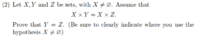# Let X, Y, Z be sets, X not empty, X-cross-Y = X-cross-Z. Prove Y = Z. Clearly show use of "X not empty"

#### Cactusguy

##### New memberI'm in an Intro to Abstract Mathematics class, and I have this proof on my homework. I'm not really sure how to do this one.
I know that X x Y consists of a set of cartesian pairs with values (a,b), where a is an element in X and b is an element in Y. X x Z consists of a set of cartesian pairs with values (a,c), where c is an element of Z. If we assume Y=Z to not be true, there must be a value of b that is not in Z. This would mean that there is a pair (a,b) where there is no value c that equals b. Therefore, X x Y could not equal X x Z if Y didn't equal Z.

That's as close as I've gotten, but it feels wordy and like I haven't really proved anything. I've basically just said "they are equal because they are". Can anyone help?

#### tkhunny

##### Moderator
Staff member
Where's your use of X not the null set?

Is there room for an Injection or Surjection in your argument?

#### pka

##### Elite Member
Do you know that $$\displaystyle \emptyset\times Y=\emptyset~?$$ If that is the case then the the conclusion fails.
Not knowing you instructor's style, I can only tell you mine. Any set theory proof should be simple and straightforward.
Thus to prove that set $$\displaystyle Y$$ equals set $$\displaystyle Z$$ one must show that $$\displaystyle t \in Y\iff t \in Z$$
There $$\displaystyle \exists s\in X$$. You must explain why the is true.
If $$\displaystyle t\in Y$$ then $$\displaystyle (s,t)\in X\times Y$$. EXPLAIN!
Does that mean $$\displaystyle (s,t)\in X\times Z~?$$ Does that mean $$\displaystyle t\in Z~?$$

What if $$\displaystyle w\in Z$$ how do you prove that $$\displaystyle w\in Y~?$$

Does that prove that $$\displaystyle Y=Z~?$$ If it does, then explain HOW & WHY.

#### frank789

##### Junior Member
bumping pkas answer down 50 iq points to my level, I once had a prof say to show two sets are equivalent, take some element t, put it in the first set, then show that it is in the other set. just in case a less mathy explanation helps.

#### Jomo

##### Elite Member
bumping pkas answer down 50 iq points to my level, I once had a prof say to show two sets are equivalent, take some element t, put it in the first set, then show that it is in the other set. just in case a less mathy explanation helps.
I doubt that your prof just said that. Let A and B be two sets. Then to show that A=B you must show that A is a subset of B and that B is a subset of A. To do this you take a in A and show it is in B (So A is a subset of B) and then you take a b in B and show that it is in A (So B is a subset of A). Combining we get A=B

NOT insulting you at all, but I bet pka's iq is more than 50 points than yours (and mine).

#### HallsofIvy

##### Elite Member
To prove that two sets, A, B, are equal (not "equivalent"- that depends on what equivalence relation you are talking about) you show that A is a subset of B, then show that B is a subset of A. And to prove that "A is subset of B" start with "If x is a member of A" then use the definition and properties of A and B to conclude "x is a member of B.

Here, "A" is Y and "B" is Z. If y is a member of Y then, for x any member of X, (x, y) is a member of X x Y. Since X x Y= X x Z, (x, y) is a member of X x Z so y is a member of Z. Therefore, Y is a subset of Z.

Now if z is a member of Z then, for x any member of X, (x, z) is a member of X x Z. Since X x Y= X x Z, (x, z) is a member of X x Y so z is a member of Y. Therefore Z is a subset of Y.

Therefore Y= Z.